# Math in Focus Grade 2 Chapter 7 Practice 4 Answer Key Comparing Lengths in Centimeters

Practice the problems of Math in Focus Grade 2 Workbook Answer Key Chapter 7 Practice 4 Comparing Lengths in Centimeters to score better marks in the exam.

## Math in Focus Grade 2 Chapter 7 Practice 4 Answer Key Comparing Lengths in Centimeters

Look at each drawing. Then fill in the blanks.

Question 1.
Which is longer? Drawing ___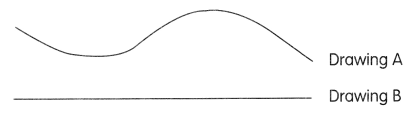Answer: Drawing A is longer than Drawing B

Question 2.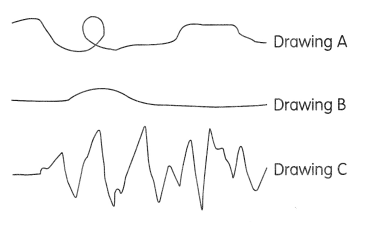Drawing ___ is the shortest.
Drawing ___ is the longest.
Answer: Drawing B is the shortest.
Drawing C is the longest.

Find each length.

Question 3.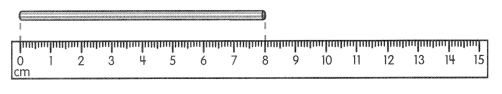The straw is about ___ centimeters long.

Question 4.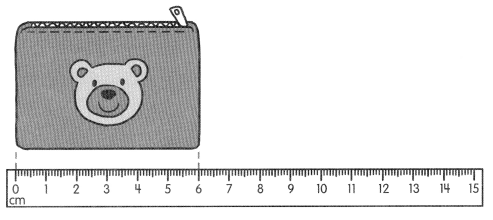The wallet is about ____ centimeters long.

Question 5.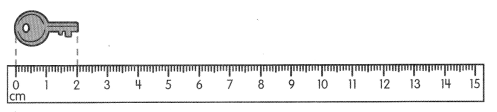The key is about __ centimeters long.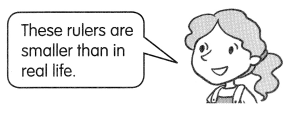Find each length.

Question 6.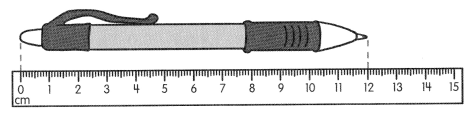The pen is about _________ centimeters long.

These rulers are smaller than in real life.Question 7.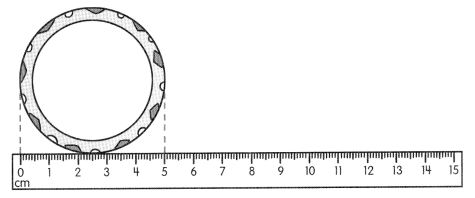The bracelet is about _________ centimeters wide.

Use your answers for Exercises 3 to 6. Fill in the blanks with longer or shorter.

Question 8.
The pen is _________ than the straw.
Answer: The pen is longer than the straw.

Question 9.
The key is _________ than the pen.
Answer: The key is shorter than the pen.

Question 10.
The wallet ¡s _________ than the straw.
Answer: The wallet is shorter than the straw.

Use your answers for Exercises 3 to 7. Fill in the blanks.

Question 11.
The straw is ___ centimeters longer than the key.
The straw is 4 centimeters longer than the key
Explanation:
Given,
Straw is 8 cm,
Key is 4 cm,
By subtracting 4 from 8 we get 4,
Therefore, the straw is 4 centimeters longer than the key.

Question 12.
The straw is ___ centimeters shorter than the pen.
Answer: The straw is 4 centimeters shorter than the pen.
Explanation:
Given,
Straw is 8 cm,
Pen is 12 cm,
By subtracting 8 from 12 we get 4,
Therefore, the straw is 4 centimeters shorter than the Pen

Question 13.
The pen is ____ centimeters longer than the key.
Answer: The pen is 10 centimeters longer than the key.
Explanation:
Given,
Pen is 12 cm,
Key is 2 cm,
By subtracting 2 from 12 we get 10,
Therefore, the Pen is 10 centimeters longer than the key

Question 14.
The bracelet is ___ centimeter shorter than the wallet.
Answer: The bracelet is 1 centimeter shorter than the wallet.
Explanation:
Given,
Bracelet is 5 cm,
Wallet is 6 cm,
By subtracting 5 from 6 we get 1,
Therefore, the bracelet is 1 centimeter shorter than the wallet

Question 15.
The longest object is the _____
Answer: The longest object is the pen with 12 cm long.

Question 16.
The shortest object is the ____
Answer: The shortest object is the key with 2 cm.